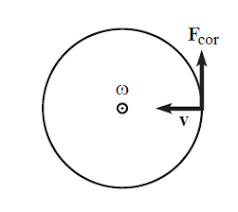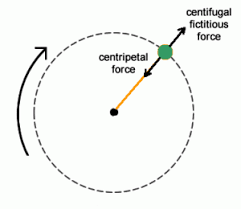## How to Calculate and Solve for Number of Revolutions per Minute and Angular Velocity of Motion of Circular Path | The Calculator EncyclopediaThe image above represent angular velocity.

To compute the angular velocity, one essential parameter is needed and its parameter is Number of Revolutions per Minute (N).

The formula for calculating angular velocity:

ω = 2πN / 60

Where;
ω = Angular velocity
N = Number of revolutions per minute

Let’s solve an example;
Find the Angular Velocity with a number of revolutions per minute as 60.

This implies that;
N = Number of revolutions per minute = 60

ω = 2πN / 60
ω = 2 x π x 24 / 60
ω = 150.816/ 60
ω = 2.5136

Therefore, the angular velocity is 2.5136 rad/s.

Calculating the Number of Revolutions per Minute when Angular Velocity is Given.

N = ω60 /

Where;
N = Number of revolutions per minute
ω = Angular velocity

Let’s solve an example;
With an angular velocity of 40. Find the number of revolutions per minute?

This implies that;
ω = Angular velocity = 40

N = ω60 /
N = 40 x 60 / 6.284
N = 2400 / 6.284
N = 381.9

Therefore, the number of revolutions per minute is 381.9 min.

## How to Calculate and Solve for Mass, Angular Velocity, Radius and Centrifugal Force of a Body | The Calculator EncyclopediaThe image above represents the centrifugal force.

To compute for the centrifugal force, three essential parameters are needed and these parameters are Mass of the body (m), Angular Velocity of the body (w) and Radius (r).

The formula for calculating the centrifugal force:

F = mω²r

Where:
F = Centrifugal Force
m = mass of the body
ω = angular velocity

Let’s solve an example;
Find the centrifugal force with mass of the body as 12, angular velocity as 32 and a radius of 8.

This implies that;
m = mass of the body = 12
ω = angular velocity = 32

F = mω²r
F = 12 x 32² x 8
F = 12 x 1024 x 8
F = 98304

Therefore, the centrifugal force is 98304 N.

Calculating the Mass of the body (m) when the Centrifugal Force, Angular Velocity and Radius is Given.

m = F / w2r

Where;
m = mass of the body
F = Centrifugal Force
ω = angular velocity

Let’s solve an example;
Find the mass of a body when centrifugal force is 140 with an angular velocity of 24 and a radius of 10.

This implies that;
F = Centrifugal Force = 140
ω = angular velocity = 24